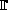# Finite field calculator

This tool allows you to carry out algebraic operations on elements of a finite field.

A finite field K=q is a field with q=pn elements, where p is a prime number. For the case where n=1, you can also use Numerical calculator.

First give the number of elements: q =

If q is not prime (i.e., n>1), the elements ofq must be described by a generator x whose minimal polynomial P(x) overp is irreducible of degree n. In this case, you should define:

P(x) =

In order to access WIMS services, you need a browser supporting forms. In order to test the browser you are using, please type the word wims here: and press Enter''.